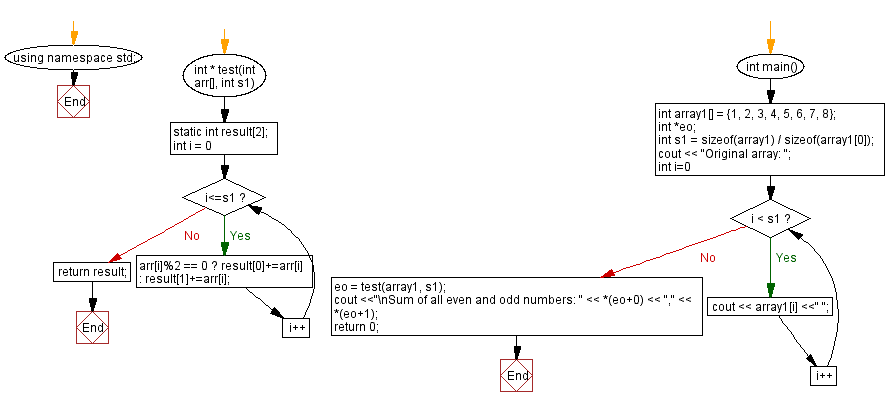﻿ C++ : Calculate the sum of all even and odd numbers in an array

# C++ Exercises: Calculate the sum of all even and odd numbers in an array

## C++ Basic: Exercise-47 with Solution

Write a C++ program to calculate the sum of all even and odd numbers in an array.

Sample Solution:

C++ Code :

``````#include <iostream>
using namespace std;
int * test(int arr[], int s1) {
static int result;
for(int i = 0; i<=s1; i++)
{
arr[i]%2 == 0 ? result+=arr[i] : result+=arr[i];
}
return result;
}
int main()
{
int array1[] = {1, 2, 3, 4, 5, 6, 7, 8};
int *eo;
int s1 = sizeof(array1) / sizeof(array1);
cout << "Original array: ";
for (int i=0; i < s1; i++)
cout << array1[i] <<" ";
eo = test(array1, s1);
cout <<"\nSum of all even and odd numbers: " << *(eo+0) << "," << *(eo+1);
return 0;
}
``````

Sample Output:

```Original array: 1 2 3 4 5 6 7 8
Sum of all even and odd numbers: 20,16
```

Flowchart:C++ Code Editor:

What is the difficulty level of this exercise?

﻿

## C++ Programming: Tips of the Day

Why is there no std::stou?

The most pat answer would be that the C library has no corresponding "strtou", and the C++11 string functions are all just thinly veiled wrappers around the C library functions: The std::sto* functions mirror strto*, and the std::to_string functions use sprintf.

Ref: https://bit.ly/3wtz2qA

We are closing our Disqus commenting system for some maintenanace issues. You may write to us at reach[at]yahoo[dot]com or visit us at Facebook